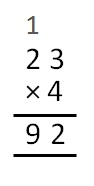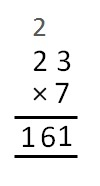Multiplication of Fractions

Chapter 2 Class 7 Fractions and Decimals
Concept wise

Let’s do this by examples.

2/3 × 4

= (2 × 4)/3

= 8/3

9 / 8 × 2

= (9 × 2)/8

= 9/4

2/3 × 5/4

= (2 × 5)/(3 × 5)

= 10/12

4 × 3 2 / 7

= 4 × (3+2/7)

= 4 × ((3 × 7 + 2)/7)

= 4 × ((21 + 2)/7)

= 4 × 23/7

= (23 × 4)/7

= 92/77/2 × 4 3/5

= 7/2 × (4+3/5)

= 7/2 × ((4 × 5 + 3)/5)

= 7/2 × ((20 + 3)/5)

= 7/2 × 23/5

= (7 × 23)/(2 × 5)

= 161/10Learn in your speed, with individual attention - Teachoo Maths 1-on-1 Class

### Transcript

Multiplication of Fractions Let’s do this by examples. 𝟐/𝟑 × 4 = (2 × 4)/3 = 8/3 𝟗/𝟖 × 2 = (9 × 2)/8 = 9/4 𝟐/𝟑 × 𝟓/𝟒 = (2 × 5)/(3 × 5) = 10/12 4 × 3 𝟐/𝟕 = 4 × (3+2/7) = 4 × ((3 × 7 + 2)/7) = 4 × ((21 + 2)/7) = 4 × 23/7 = (23 × 4)/7 𝟕/𝟐 × 4 𝟑/𝟓 = 7/2 × (4+3/5) = 7/2 × ((4 × 5 + 3)/5) = 7/2 × ((20 + 3)/5) = 7/2 × 23/5 = (7 × 23)/(2 × 5) = 161/10